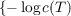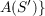#### Vol. 49, No. 2, 1973

 Download this articleFor screen For printingRecent Issues Vol. 325: 1 Vol. 324: 1  2 Vol. 323: 1  2 Vol. 322: 1  2 Vol. 321: 1  2 Vol. 320: 1  2 Vol. 319: 1  2 Vol. 318: 1  2Online Archive Volume: Issue:The Journal Subscriptions Editorial Board Officers Contacts Submission Guidelines Submission Form Policies for Authors ISSN: 1945-5844 (e-only) ISSN: 0030-8730 (print) Special Issues Author Index To Appear Other MSP Journals
Almost isometries of Banach spaces and moduli of planar domains

### Richard Rochberg

Vol. 49 (1973), No. 2, 445–466
##### Abstract

Let C(X) and C(Y ) be the supremum normed Banach spaces of continuous complex valued functions on the compact Hausdorff spaces X and Y respectively. Let A and B be closed subspaces of C(X) and C(Y ) respectively. A map from A to B will mean a continuous invertible linear map of A to B. The set of all such maps will be denoted by L(A,B). For T in L(A,B) define c(T) = 1(T∥∥T1). A generalization of the Banach-Stone theorem is proved which shows that there is a constant d < 1 such that if A and B satisfy certain additional technical restrictions and there is a T in L(A,B) with c(T) > d then X and Y are homeomorphic. Furthermore, T is, roughly, composition with this homeomorphism.

For S a connected subset of C bounded by a finite number of disjoint Jordan curves, denote by A(S) the Banach space of functions in C(S) which are analytic on the interior of S. For two such domains, S and S, set d(S,S) = inf;T a linear map of A(S) onto. By analyzing maps T for which c(T) is nearly one, it is shown that d(,) is a metric on the space of moduli of such domain (considered as Riemann surfaces) and that this metric induces the classical moduli topology.

Primary: 30A98
Secondary: 46E15
##### Milestones